## Sunday, 19 May 2013

### A number theory problem from online games Part 1

The situation comes from online game in a very natrual style.

Unlike modern banking system, the statistics (like money) in online games are usually integer, but that makes some problem (to the producer sometimes, and sometimes to players) in particular the statistic conversion.

Like, we are obtaining energy A from energy B at a rate of 36.5%. It is natrual that it takes 365 units of B for us to get 1000 units of A. For what if we want 1 units of A? 3 units?

In the game that I am playing they take the rounding off approach --- of course --- this is beneficial if we goes B->A but not-so-good when we go in another way. For example 1 unit of A is supposed to take 0.365 units of B and now it's rounded off to zero. 3 units of A takes 1 unit of B to convert with. Of course, the system won't let you to obtain 1 unit of A 'with zero unit of B' --- you need at least one unit of input.

The problem comes: in what ratio (in particular, the amount of A I want every time) so that we maximize our gain (i.e. ratio above the supposed rate)? We can now state our problem:

Instance: A real number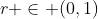Prroblem: Find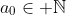to maximize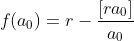.

Well, r causes a bit of trouble when it isn't rational, so we modify the instance a bit:

Instance: A sequence of real number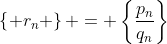that converges to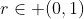. It follows that the sequence is an approximation to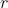that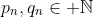and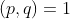. Moreover, it follows that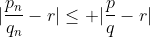for all fractions that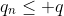. If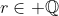, the sequence constantly equal to the fraction that equals to.

We should also specify that we can't convert to something (larger than zero) using zero resources. So the problem becomes:

Problem: Find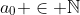to maximize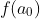as defined above, with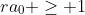.

Where should we start with? It is clear that when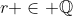the problem can be easily solved. To see this we shall consider rational instance for the rest of (this part of) the passage with the notation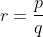.

Lemma. For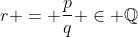,.

Proof. For, there is a multiplicative inverse of p mod q. Let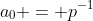in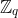, it is clear that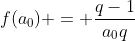.

For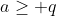,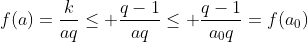.

It's like that the above lemma gives us a possible answer to the problem, but is it the best answer? Let's divide it in two cases:

Theorem. If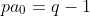, then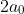is the optimal answer.

Proof. It is clear that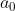can't be a valid answer, and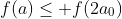for all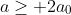using similar arguement with the lemma. To see thatfor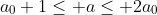, notice that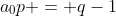so that the integer part of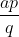do not increase in the given range, the result immediately follows.

Similarly, if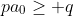we check all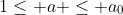to get the optimal answer. It follows that the optimal answer is at mostusing the similar arguement.

If we want to be faster, check all crossing and the corresponding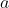value so that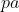is just under the multiple of q, now we should get the answer.

Why 'should'? Think about the situation:

Suppose we getas the 'optimal' answer using the faster way, is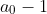a better answer? Is this even possible?... We shall see it shortly...